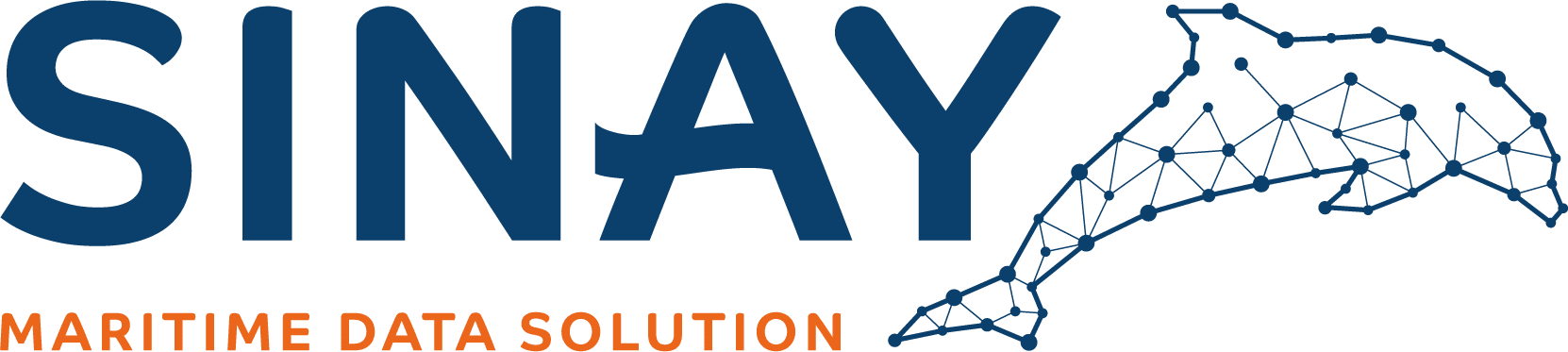# How Is ETA Computed Using Artificial Intelligence?

Estimated time of arrival (ETA) computations, specific to ports and ships, determine when a vessel is expected to arrive to a port. The computations also enable vessels to be tracked during their entire voyage.
[ no_toc ]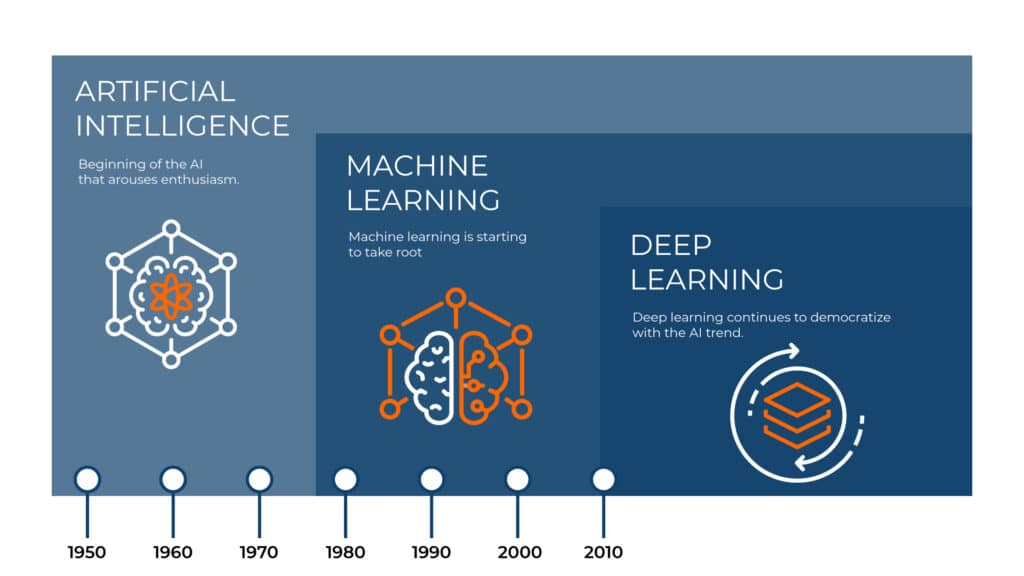## Table of content

You may also like…

What is the meaning of ETA, ETD, ATA, and ATD in shipping

This article will introduce how to compute estimated time of arrival (ETA) of a vessel to its port destinationThe ETA computations explained are to help ports and the shipping industry understand how ETA is computed and how important a precise ETA is for their business activitiesETA is one of the most influential factors for maritime supply chain efficiency.

## What Is ETA for Ports and Ships?

computations, specific to ports and ships, determine when a vessel is expected to arrive to a port. The computations also enable vessels to be tracked during their entire voyage. A precise ETA calculation is not easy to determine and requires many factors and variables

## How can AI enhance Your ETA Predicions ?

Read more about how to reduce turnaround time and optimize port calls thanks to smart ETA predictions.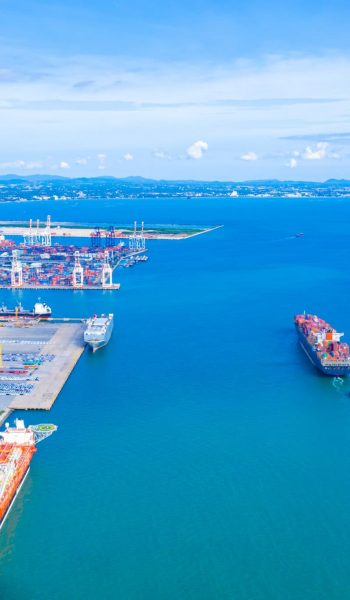## How Is ETA Computed?

ETA may be computed with a standard method or an Artificial Intelligence (AI) method.

ETA computations are based on the following formula:

f(x) = y

When;

f = function, the transformation of data

x = data

y = ETA

Thus, ETA is based on data, and the data that is plugged into this formula are the most important variables.

The data used when calculating ETA with AI come from ship messages, satellites, and radio signals. This could help determine, for example, geographic positioning. This data allows AI algorithms to find the optimal route and expected time of arrival at a port.

### Standard Calculation

The standard calculation to determine ETA is,

*ETA = Distance/Speed

*This is the base of ETA and the most standard calculation that exists, and therefore is not how Sinay computes ETA. Rather this is described to understand the complex explanations for computing ETA with Artificial Intelligence.

Considering f(x)=y, when using the standard method, f is only given two features*, distance and speed. This is an extremely simplified way to compute ETA.

*a feature, also known as a variable, can consist of variables including vessel characteristics (cargo, tanker, size), geographical positioning, speed, and environmental events (weather, currents)

In this calculation the change of speed of a vessel cannot be accounted for; therefore, it is not precise enough to predict an accurate ETA. This is because on a vessel’s journey the speed is constantly changing. A vessel never travels the same speed the entire route due to environmental aspects that must be considered.

## Artificial Intelligence and ETA

This article will focus on measuring ETA with AI statistics and AI machine learning (ML). Statistics dates to the 1600s. Modern Artificial Intelligence traces back to the 1950s, and thus it has been around longer than one may imagine. Already in 1997 computer Deep Blue beat Kasparov, a world-champion chess player, in a match.

Artificial Intelligence (AI), of which machine learning (ML) is a part of, and derived from statistics, is one method to obtain a more accurate prediction of ETA. AI is composed of many different models. As demonstrated in the image below, statistics encompasses Artificial Intelligence which encompasses machine learning.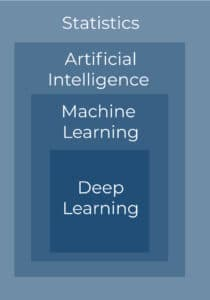Both AI statistics and AI ML use real-time and historical data. ML, apart, is complex because it can learn patterns. It can therefore compute real-time ETA based on historical examples. For example, a boat of a certain height and width traveling at a certain speed with a certain amount of wind would be recorded by a ML model, the model would learn from this, then predict future ETA real-time schemas. The model learns from historical data like a child learns how to put a puzzle together. The child remembers shapes from historical data which helps them to put the right shape in the right place in real-time. Essentially, the difference between AI statistics and AI ML is that ML can learn from itself.

This does not mean that AI ML is better for every case, but for ETA it is the best method since it can handle more complexity.

## AI Statistics

A common form of AI statistics which can compute ETA is linear regression.

Considering f(x) = y, when linear regression is used, it can compute ETA as a continuous variable (for example, changing speed and remaining distance). This makes linear regression better than the standard calculation, as with the standard calculation speed and distance is unchanging.

Linear regression can deal with several features but depending on how the features interact with each other, it determines if linear regression can be used. If features are complex, linear regression will not give an accurate computation because it cannot see complex links between features. Therefore, since ETA is composed of complex features, linear regression alone should not be used.

Figure 1 shows the difference between a linear and a non-linear function.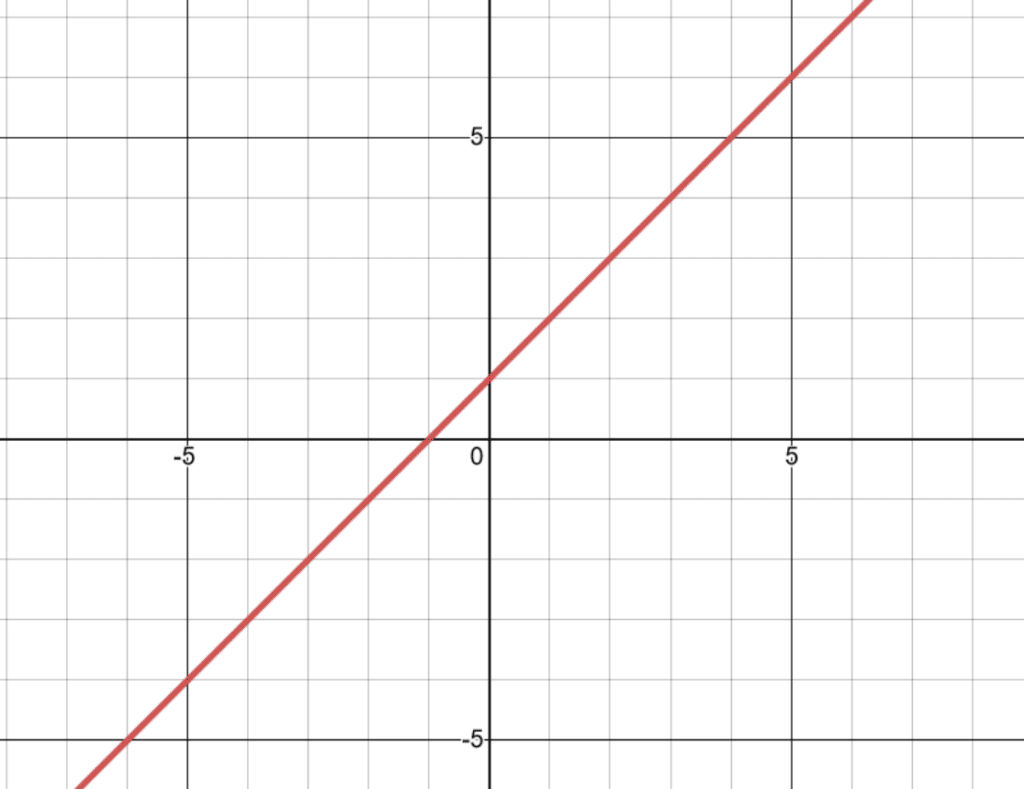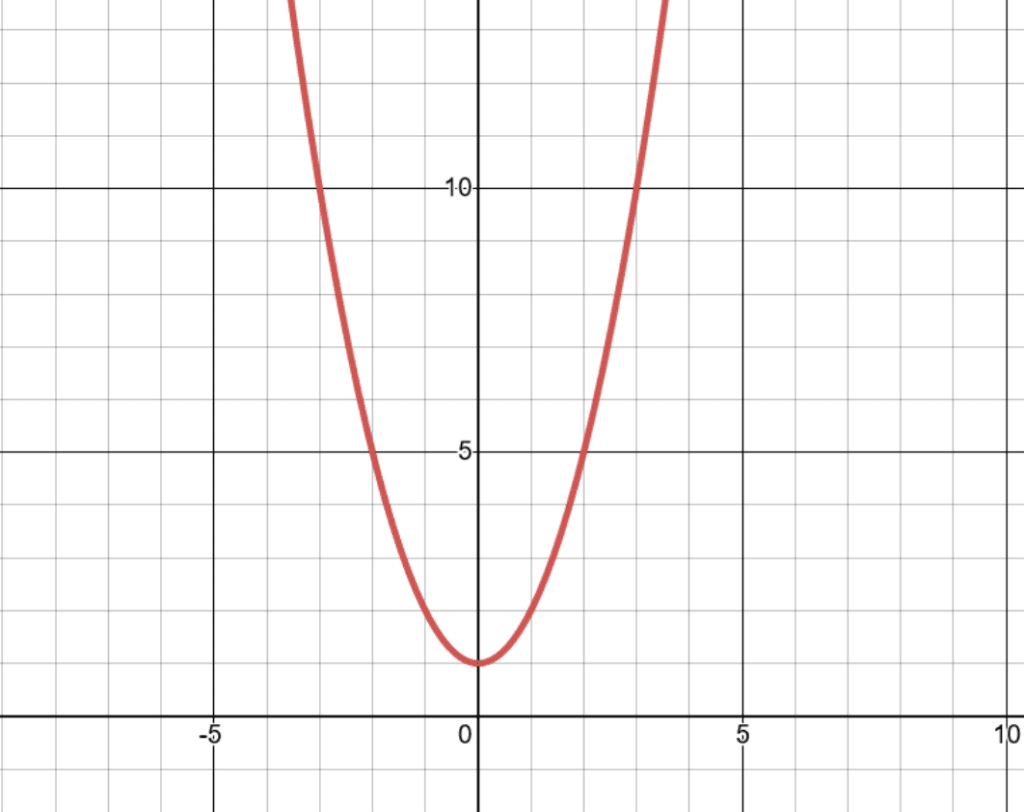Figure 1– Linear (left) vs Non-Linear (right) Function

Linear Function:f(X)=aX+bfX=aX+b

Non-Linear Function:f(X)=aX2+bX+cfX=aX2+bX+c

### What Is a Complex Feature?

A feature is a piece of information about a situation at hand, like the speed of the vessel or the remaining distance before reaching the destination port. Those can be used with linear regression because they are continuous, straightforward values.

However, features can be more complex, such as the effect of weather on the vessel’s speed which affects time of arrival. Weather can be composed of winds and currents, themselves composed of velocity field, wave height, wave frequency, etc. Thus, the weather cannot be represented using a linear function and needs machine learning to approximate the non-linear function that characterizes the weather and uses it to predict its effect on speed and time of arrival.

Regarding the distance to the port of destination, captains choose a trajectory as a car driver must choose a highway. As there are different highways on the sea (maritime lanes), the highway chosen by the captain will not result in the same distance to the port or the same weather along the way. The choice of the route will thus have a significant impact on the ETA.

To sum it up, if ETA were only speed and distance, then linear regression could be used. However, the speed is affected by changing features. We cannot say that if it is sunny the ship will go 10mph since there are many other factors interacting with it. During a voyage, there can be wind, rain, sun, and different currents. When interdependent features like this move and change, machine learning must be used along with statistical models to handle all the data. The machine learning model can also be programmed to take into the account the trajectory that the captain will possibly follow (the route) to have the most precise ETA as possible. As mentioned above, different trajectories produce different distances and weather patterns that affect the ETA.

## How can AI enhance Your ETA Predicions ?

Read more about how to reduce turnaround time and optimize port calls thanks to smart ETA predictions.## AI Machine Learning

Machine learning (ML) can be seen as the next step of AI statistics. The base of AI is statistics, then machine learning uses more recent algorithms, adapting to specific use cases.

Considering f(x) = y, f is more complex when using ML. ML can approximate nonlinear functions (f) that represent the behavior of the complex data and can thus be used to predict future values.

ML algorithms can process a lot of data and learn to recognize links and connections to see how different variables will impact one another. The more data that can be used the easier it is for the algorithms to learn (until a certain point if there is too much data there may be computation time issues), and the more complex the prediction is the more data is needed. This enables the algorithms to account for how external variables will affect speed and distance. Most importantly, ML can learn time and cycles. If ML algorithms learn on several years of data, they can make advanced calculations, such as how certain seasons may affect the ETA of a vessel or ship.

## The Benefits of Using AI for ETA

Ports, shipping companies, and Beneficial Cargo Owners can have a more precise ETA using AI algorithms.

A precise ETA will impact supply chains and is part of a larger schema that can help reduce port congestion, increase route optimization, and optimize route planning.

In fact, calculating ETA is the first step to be able to create models for port congestion, route planning, and route optimization models.

ETA also helps with resource planning, which is having the right person at the right place at the right time.

Precise ETAs help ports to minimize vessel turnaround time, help shipping companies to track their vessels, and help BCOs to know where their cargo is located. Ports can also know exactly how long it will take ships to come from the waiting zone to the dock, enabling them to be more prepared for the docking process.

## Sinay ETA Module and Artificial Intelligence

Sinay developed advanced algorithms to create the ETA Module software using AI, including statistics and Machine Learning, that can predict the ETA, the optimized route, and the remaining route distance for commercial ships and vessels.

Sinay’s skilled AI experts know how to take the large amounts of data received and clean them to use them with powerful AI algorithms. The models learn how to compute ETA on this clean dataset, where the historical ETA is known. The model is then able to compute ETA and vessel routes in real-time. The historical data is what the model learns from, while the real-time data is what the model is given to compute ETA and routes.

Overall, using machine learning to compute ETA and route planning is complex but very important for maritime activities.